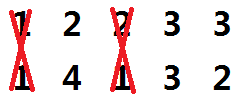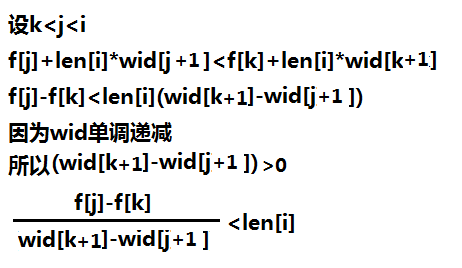# bzoj1597 [Usaco2008 Mar]土地购买（斜率）

Description

Input

Output

Sample Input
4
100 1
15 15
20 5
1 100

Sample Output
500

HINT
FJ分3组买这些土地: 第一组:100x1, 第二组1x100, 第三组20x5 和 15x15 plot. 每组的价格分别为100,100,300, 总共500.

f[i]=min{f[j]+max(len[j]~len[i])*max(wid[j]~wid[i])}

ta没有要求一定要按照顺序购买

# 直接把这一块删掉• 这块土地如果和ta旁边的土地一起购买的话
ta的长宽对价格是没有影响的
• 如果单独购买的话，购买排在ta前后的土地还要花钱
单独购买花的钱就要多于分组购买了

f[i]=min{f[j]+len[i]*wid[j+1]}1.维护双端队列
2.转移时从队首转移：队中依次有a,b,c等元素，

3.入队时：队中依次有a,b,c等元素，待入队元素为d

# tip

WA了几次。。。STO

这里写代码片
#include<cstdio>
#include<cstring>
#include<iostream>
#include<cmath>
#include<algorithm>
#define ll long long

using namespace std;

const int N=50010;
ll f[N];
int n,tot=0,q[N],tou,wei;
struct node{
ll x,y;
};
node b[N],a[N];

int cmp(const node &a,const node &b)
{
if (a.x!=b.x) return a.x<b.x;
else return a.y>b.y;
}

double get(int j,int k)
{
double x1=(double)f[j]-f[k];
double x2=(double)a[k+1].y-a[j+1].y;
return (double)x1/x2;
}

void doit()
{
tou=wei=0;
int i,j;
for (i=1;i<=n;i++)
{
while (tou<wei&&get(q[tou+1],q[tou])<a[i].x) tou++;
f[i]=f[q[tou]]+a[i].x*a[q[tou]+1].y;
while (tou<wei&&get(i,q[wei])<get(q[wei],q[wei-1])) wei--;
q[++wei]=i;
}
printf("%lld",f[n]);
}

int main()
{
scanf("%d",&n);
for (int i=1;i<=n;i++)
scanf("%lld%lld",&b[i].x,&b[i].y);
sort(b+1,b+1+n,cmp);
for (int i=1;i<=n;i++)
{
while (tot&&b[i].y>=a[tot].y) tot--;
tot++;
a[tot]=b[i];
}
n=tot;
doit();
return 0;
}
posted @ 2017-09-25 18:27  wtt3117  阅读(97)  评论(0编辑  收藏  举报